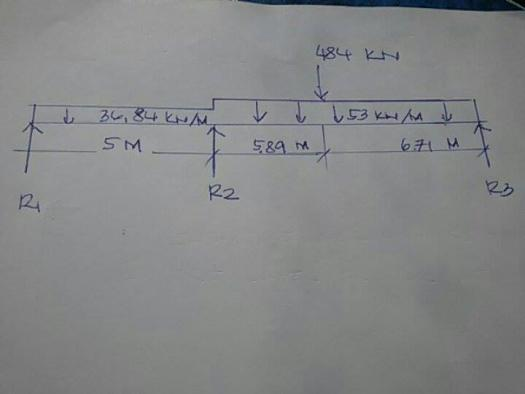# Continues beam### Re: Continues beam

Compute reactions and maximum moment

### Re: Continues beam

no one can solve?### Re: Continues beam

$M_1L_1 + 2M_2(L_1 + L_2) + M_3L_2 + \dfrac{6A_1\bar{a}_1}{L_1} + \dfrac{6A_2\bar{b}_2}{L_2} = 0$

$M_1 = M_3 = 0$

$L_1 = 5 ~ \text{m}$

$L_2 = 5.89 + 6.71 = 12.6 ~ \text{m}$

$\dfrac{6A_1\bar{a}_1}{L_1} = \dfrac{w_oL^3}{4} = \dfrac{36.84(5^3)}{4}$

$\dfrac{6A_1\bar{a}_1}{L_1} = 1151.25 ~ \text{kN}\cdot\text{m}^2$

$\dfrac{6A_2\bar{b}_2}{L_2} = \dfrac{w_oL^3}{4} + \dfrac{Pb}{L}(L^2 - b^2) = \dfrac{53(12.6^3)}{4} + \dfrac{484(6.71)}{12.6}(12.6^2 - 6.71^2)$

$\dfrac{6A_2\bar{b}_2}{L_2} = 55,820.32 ~ \text{kN}\cdot\text{m}^2$

Thus,
$0 + 2M_2(5 + 12.6) + 0 + 1151.25 + 55,820.32 = 0$

$M_2 = -1618.51 ~ \text{kN}\cdot\text{m}$

First Span

$V_1 = V_2 = \frac{1}{2}(36.84)(5) = 92.1 ~ \text{kN}$

${R_1}' = {R_2}' = \dfrac{1618.51}{5} = 323.702 ~ \text{kN}$

Second Span

$V_2 = \frac{1}{2}(53)(12.6) + \dfrac{484(6.71)}{12.6} = 591.65 ~ \text{kN}$

$V_3 = \frac{1}{2}(53)(12.6) + \dfrac{484(5.89)}{12.6} = 560.15 ~ \text{kN}$

${R_2}' = {R_3}' = \dfrac{1618.51}{12.6} = 128.453 ~ \text{kN}$

Reactions
$R_1 = 92.1 - 323.702 = -231.602 ~ \text{kN}$

$R_2 = 92.1 + 323.702 + 591.65 + 128.453 = 1135.905 ~ \text{kN}$

$R_3 = 560.15 - 128.453 = 431.697 ~ \text{kN}$

Maximum moment will occur at the point of application of concentrated load
$M_{max} = 431.697(6.71) - 53(6.71)\left( \dfrac{6.71}{2} \right)$

$M_{max} = 1703.55 ~ \text{ kN}\cdot\text{m}$

## Add new comment

### Deafult Input

• Allowed HTML tags: <img> <em> <strong> <cite> <code> <ul> <ol> <li> <dl> <dt> <dd> <sub> <sup> <blockquote> <ins> <del> <div>
• Web page addresses and e-mail addresses turn into links automatically.
• Lines and paragraphs break automatically.
• Mathematics inside the configured delimiters is rendered by MathJax. The default math delimiters are $$...$$ and $...$ for displayed mathematics, and $...$ and $...$ for in-line mathematics.

### Plain text

• No HTML tags allowed.
• Lines and paragraphs break automatically.